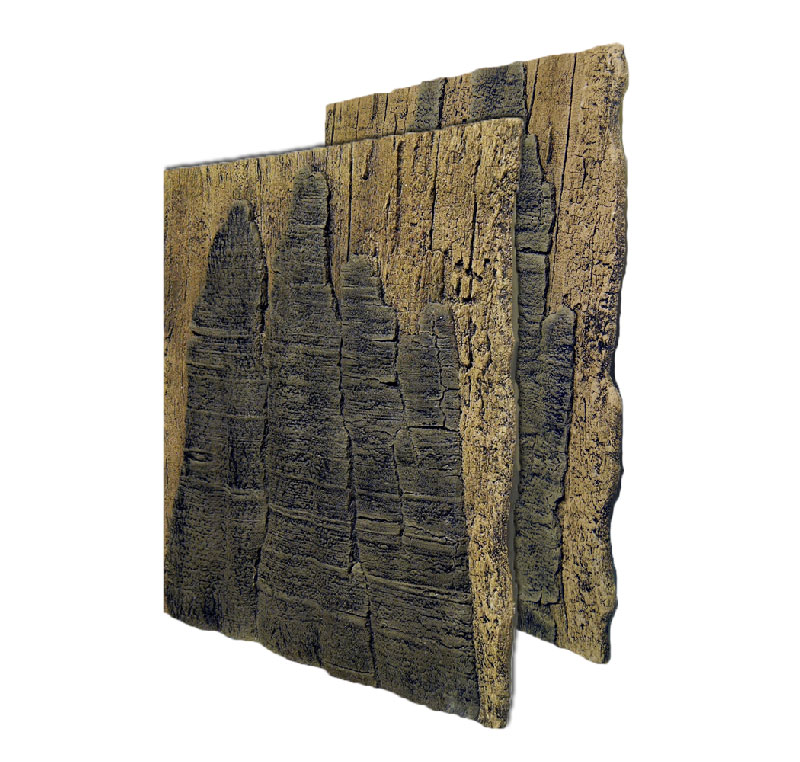# Rainforest Slimline aquarium backgroundsTank (WxH) Slimline Formula 50×50/45 A50 70×50/45 B50+C50 100×50 A50+B50 120×50 A50+B50+C50 150×50 B50+A50+B50 170×50 B50+A50+B50+C50 200×50 B50+A50+B50+A50
 Tank (WxH) Slimline Formula 50×70 A70 100×70 A70+B70 120/130×70 A70+B70+A70 150×70 B70+A70+B70 160/170×70 B70+A70+B70+A70 180/200×70 B70+A70+B70+A70 250×70 B70+A70+B70+A70+B70 300×70 B70+A70+B70+A70+B70+A70
 Tank (WxH) Slimline Formula 50×60 A60 70×60 B60+C60 100×60 A60+B60 120×60 A60+B60+C60 160×60 B60+A60+B60 170×60 B60+A60+B60+C60 200×60 B60+A60+B60+A60
[ts_icon heading=”5 COLOURS FOR ANY TANK AND TASTE” size=”small”][/ts_icon]

You can choose among 5 different colours which are available for all ARSTONE products:

• Basalt Gneiss
• Grey Gneiss
• Red
• White
• Alpenkalk
[ts_icon heading=”Slimline backgrounds in tanks”][/ts_icon][slupy_portfolio model=”2″ filterable=”off” pagination=”none” image_size=”large” ids=”79″]
Wir verwenden Cookies. Durch die weitere Nutzung der Seite stimmst du der Verwendung von Cookies zu.